# Comb-drive actuator design

We consider the case depicted in Figure 1 of a comb-drive actuator. This actuator is the favored electrostatic actuator for its relative large range and good force/electrical power ratio. It is composed of N fingers on the rotor that are interleaved with the N+1 fingers of the stator.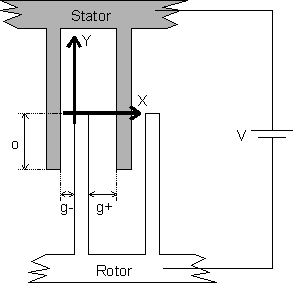Figure 1: View of one period of an electrostatic comb-drive actuator

We want to know the force attracting the electrodes when we apply a voltage. We will obtain this force via the differentiation of the expression of the energy stored in the system.

## I Electrostatic force

In order to derive the mechanical force generated by the electrostatic actuator we will differentiate the work of the force i.e., the energy stored in the system. The energy stored in a capacitor is given by: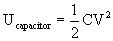(1)
The total energy stored in the circuit is given by the energy stored in the capacitor minus the energy taken from the power source to charge it, that is: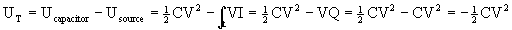(2)
We simplify the computation of the capacitance by considering only the lateral surfaces of neighbor fingers. We neglect the interaction with the part of the finger that is not engaged and with the horizontal surfaces (the fingers are considered infinitely thin). Moreover, we neglect the presence of the other fingers and the effects arising at the comb edge. Using this simplification, we may compute the capacitance of the comb by summing N times the capacitance of one finger. We describe a finger as two planar capacitors in parallel (one per side), and neglecting the fringing fields, we have: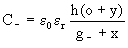(3)
and: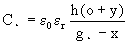(4)
where the variable and constant are shown in Figure 2.
Hence the capacitance of the comb is:
C = N Cfinger = N (C- + C+)(5)

### I.1 Longitudinal force (i.e., parallel to the finger)

This force may be assessed by: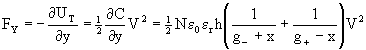(6a)
if the two lateral gaps are equal, g+ = g- = g, and x = 0, we may simplify this expression and obtain: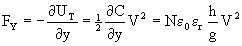(6b)

### I.2 Lateral force (i.e., perpendicular to the finger)

This force is given by: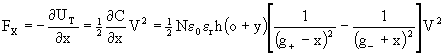(7)

If the two lateral gaps are equal and x = 0, the two lateral force are equal and the resultant net force along the X-axis is nearly zero. However, when x is different from zero, this lateral force may lead to instability when it increases more rapidly than the restoring lateral elastic force, bringing finger into contact. In a similar way, this effect may bend thin and long fingers causing short-circuit. This effect will be described in the next section.

To be complete, if there is only one side of the finger experiencing the electrostatic force, as in a gap-closing actuator, the expression simplifies slightly and yields: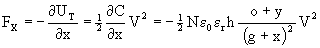(8)

## II Displacement

The comb-drive principle seems to allow displacement only limited by the length of the finger. Actually, for any voltage greater than zero there will be a longitudinal force that will pull the rotor toward the stator continuously. This may be true in some extent for a guided slider, provided a scheme for biasing has been found and the electrostatic force overcomes the friction force. However, these two later points are generally solved by suspending the rotor above the substrate, limiting the achievable displacement. Actually, the suspension creates mechanical force acting against the displacement and the net displacement is obtained when the electrostatic force is equal to this mechanical force. The suspension presents the benefit to bring the rotor to its original position when the voltage (i.e., the electrostatic force) is decreased to zero. Thus, the displacement is limited by the maximal electrostatic force that may be obtained, which in turn is limited by the maximum voltage that can be applied. We will further see that the suspended rotor is subject to lateral instability that limits this voltage, much before the ionization of the insulator between the rotor and the stator appear.

### II.1 Limitation imposed by the suspension

The suspension of the rotor creates a force FmY acting against the displacement along Y-axis that is given by: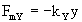(9)
where kY is the spring constant of the suspension along the Y axis (i.e., the linear elastic constant), the suspension being not deformed when y = 0. Then the static displacement of the rotor is obtained by writing the equilibrium of the mechanical and electrostatic forces. Taking the case g+ = g- = g, and er=1 (air) we have: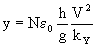(10)

Example 1:
With an air gap of 2 µm width, electrodes height of 2 µm and 10 µm of engaged electrodes we have:
FY = N* 8.85·10-12 * 2·10-6 / 2·10-6 = N*8.85·10-12 N/V2
thus it is about 0.008 µN by finger for an applied voltage of 30 V.
For the first application of the movable mirror we need a displacement of the order of l/2 (~0.5 µm). For a suspension with a spring constant of 1 N/m and with an applied voltage of 30V, we need at least 60 fingers. To obtain the displacement of several µm needed for the second application, we may either increase the number of finger or decrease the spring constant. With the same actuator and a spring constant of 0.16 N/m, the actuation range becomes 3 µm.
For this voltage, the force generated by the actuator will be 0.48 µN. For example this correspond to an acceleration of 100 g applied on a mass of 0.5·10-9 kg. This value is a good estimate of the maximum range obtained when the actuator is  used as a force feedback in an accelerometer, provided the actuator is not deflected in the reference position. In that latter case the maximum available force will be decreased by the reaction of the suspension at the 'rest' position. To account for random direction acceleration, a two-way actuator should be used.

### II.2 Limitation imposed by lateral electrostatic instability

At first thought it seems that increasing the voltage on the actuator will increase the displacement continuously. However, for a high enough voltage the lateral electrostatic force increases more rapidly than the restoring lateral elastic force when the finger moves laterally. At this point any small initial lateral displacement will inexorably bring neighbor fingers into contact. This critical voltage is formally given by the comparison between the x-derivatives of the lateral electrostatic force (i.e., Fx/ x) and the x-derivatives of the lateral mechanical force (i.e., the spring constant along X, kX):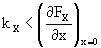(8)

Taking the case g+ = g- = g, and er=1 (air) this relationship becomes: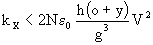(9)

Thus, the maximum voltage, Vmax, that may be applied to the actuator before instabilities occur is: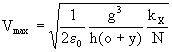(10)

We see that this limit decreases with smaller gap and with larger electrodes total lateral surface (i.e., N h (o + y)).

Example 1:
With an air gap of 2 µm width, electrodes height of 2 µm and 10 µm of engaged electrodes we have a lateral force on each surface, determined using (8):
FX = -.5 * 8.85·10-12 * 2·10-6 *10·10-6 / 4·10-12 = 2.21·10-11 N/V2
thus it is about 0.02 µN for an applied voltage of 30 V
For a full rotor finger, with the same gap on both side, the same force apply on each lateral surface, therefore in the ideal case, neither a bending moment nor a lateral force should appear as can be computed from (7). However, this equilibrium is unstable as soon as the voltage exceeds the critical voltage. To compute this voltage it is necessary to observe that the lateral stiffness of the suspension is much lower when the suspension is deflected. Thus for y=0, a typical folded beam suspension with 300 µm-long beams has a spring constant of kX = 2Ehw/l = 4533 N/m . However when y>0, the spring constant becomes kX = 5.5 E h w3/Lx2, thus kX = 503 N/m for a 10 µm deflection and kX = 125 N/m for a 20 µm deflection. The corresponding critical voltages for a 60-fingers comb-drive are, respectively:
Vmax =  1306 V, 308 V, and 124 V.
It is apparent that this limitation becomes a real problem for actuator with large range of displacement.

## III References

W. Tang, T. Nguyen, and R. Howe, "Laterally driven polysilicon resonant microstructures", Sensors and actuators, Vol. 20, pp. 25-32, 1989

W. C. Tang, "Electrostatic comb-drive for resonant sensor and actuator applications", PhD thesis, University of California, Berkeley, 1990

R. Legtenberg, A. Groeneveld and M. Elwenspoek, "Comb-drive actuators for large displacements", J. Micromech. Microeng. Vol. 6, pp. 320-329, 1996